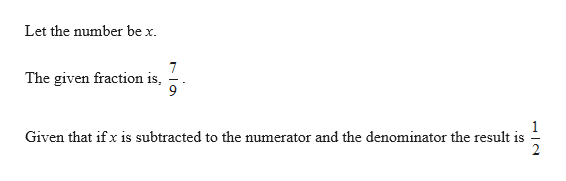# if the same # is subtracted from the numerator and denominator of 7/9, the result is 1/2 find the #

Question
12 views

if the same # is subtracted from the numerator and denominator of 7/9, the result is 1/2 find the #

check_circle

Step 1help_outlineImage TranscriptioncloseLet the number be x 7 The given fraction is Given that ifx is subtracted to the numerator and the denominator the result is fullscreen

### Want to see the full answer?

See Solution

#### Want to see this answer and more?

Solutions are written by subject experts who are available 24/7. Questions are typically answered within 1 hour.*

See Solution
*Response times may vary by subject and question.
Tagged in

### Numbers